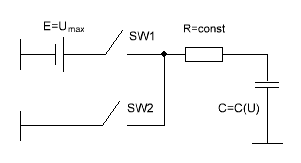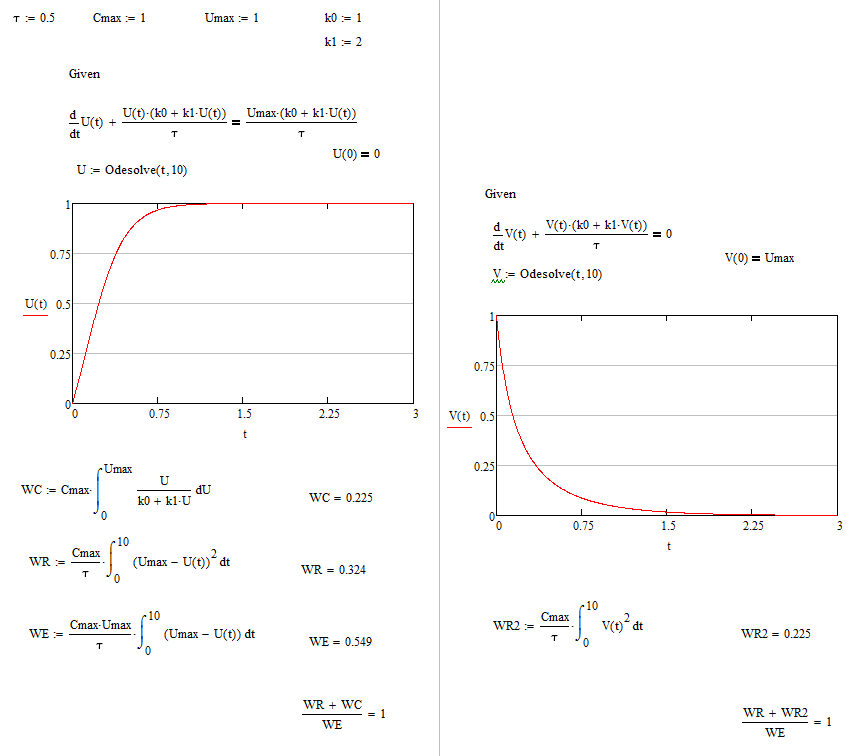Research website of Vyacheslav Gorchilin
2016-08-01
2. Parametric changing capacitance in an RC circuit. Example
In the previous part of the narrative we have proved the impossibility of further increase of energy in the RC and RL-circuit even if the resistance is parametric. Here we show that, under normal conditions, in the case of parametric capacity increase not get. According to the classification here will be considered the generator of the first kind of first order with a full cycle.
Consider the circuit of a RC-chain in which the resistance $$R$$ is a constant, and capacitance $$C$$ depends on voltage. The working scheme is simple: the first time ($$T_1$$) is closed, the key SW1 and charges the capacitor. The second time vremneni ($$T_2$$), the key SW1 is opened, but closes SW2 and the capacitor is discharged by the resistance.These two points and will be considered separately.
The General approach to the theory of transient processes described in reference . Based on this make the equation for the moment $$T_1$$: ${dU(t) \over dt} + {U(t) \over C(U) \, R} = {U_{max} \over C(U) \, R} \qquad (2.1)$ where: $$U(t)$$ is the voltage across the capacitor is $$C$$ whose capacity depends on the voltage $$C = C(U)$$, $$U_{max}$$ is the voltage at the voltage source $$E$$. Parametric dependence of the capacitance on voltage will be the fact that in this simple form: $C(U) = {C_{max} \over k_0 + k_1 \, {U(t) \over U_{max}}}, \quad U(t) \in [0, U_{max}] \qquad (2.2)$ where: $$C_{max}$$ — maximum capacity with minimal voltage $$U = 0$$, and $$U_{max}$$ — maximum voltage with a minimum capacity that is equal to the voltage at $$E$$ in range $$[0, U_{max}]$$, our parametric capacity and it will work. The coefficients $$k_0$$ and $$k_1$$, define the character of the dependence of $$C$$ from $$U$$.
The analytical solution obtained by substituting (2.2) into (2.1) the differential equation is quite cumbersome, so we use another achievement of civilization — mathematical editor,which can accurately find its solution in a visual numerical form .
For convenience, we introduce the time constant $$\tau = R\,C_{max}$$, we write the equation according to the rules of MathCAD , we substitute there for example $$\tau=0.5, \, k_0=0.2, \, k_1=4, \, U_{max}=1$$ and look at the result. As you can see, the curve of a charging capacitor is very different from the classic, when the capacity is constant. Full program of calculation in MathCAD for this example can be downloaded here.
Not to be confused with $$U(t)$$ will choose for the second time ($$T_2$$) another function $$V(t)$$ and also make the equation: ${dV(t) \over dt} + {V(t) \over C(V) \, R} = 0 \qquad (2.3)$Again, for example, substitute into the equation the same data, look at the result and compare them. Paying attention to the time axis $$t$$ we see that the parametric discharge of the capacitor lasts longer than its charge.
Knowing the voltage of you can proceed to the calculation of energies, then we will be able to compare them and to draw conclusions about an additional gain. When charging, the energy from the power source $$W_E$$ is spent on heating of the resistance $$W_R$$ and the charge of the capacitor is $$W_C$$. Next, we write these energies as separate formulas. They all follow from the classical electrical engineering. $$W_E$$ is like $$\int E {U_R \over R} \, dt$$, where $$U_R$$ is a voltage at $$R$$ : $W_E = {U_{max} \over R} \int_0^{\infty} (U_{max}-U(t)) \, dt \qquad (2.4)$ $$W_R$$ derived from the formula $$\int {U_R^2 \over R} \, dt$$ : $W_R = {1 \over R} \int_0^{\infty} (U_{max}-U(t))^2 \, dt \qquad (2.5)$ $$W_C$$ is the formula of finding the potential energy $$\int C \, U \, dU$$ : $W_С = C_{max} \int_0^{U_{max}} {U \over k_0 + k_1\,U} \, dU \qquad (2.6)$ Thus, the ratio of the received energy to the expended and needs to show us, is there any increase of energy in the first measure: ${W_R + W_C \over W_E} = K \qquad (2.7)$ Also, we can calculate the increase in energy in the full cycle: charge-discharge. It needs to lay down energy on the resistance during the discharge of the capacitor is $$W_{R2}$$ with the charge $$W_R$$, and again compare with the cost: ${W_R + W_{R2} \over W_E} = K_2 \qquad (2.8)$ $$W_{R2}$$ we find from the formula $$\int {V_R^2 \over R} dt$$ : $W_{R2} = {1 \over R} \int_0^{\infty} V(t)^2 \, dt \qquad (2.9)$
Now write down all of this in Mat. the editor and look at the results.Recommend to watch it directly in MathCAD, but the conclusion is clear: the coefficients of $$K$$ and $$K_2$$ is equal to one for all values of $$\tau, k_0, k_1$$ (within reason of course). Moreover, the result does not change even if is based on (2.2) to choose any other, for example, power law: $C(U) = {C_{max} \over \left( k_0 + k_1 \, {U(t) \over U_{max}} + k_2 \, \left({U(t) \over U_{max}}\right)^2 \right)^2}$